# Intermediate Geometry : How to find an angle in a hexagon

## Example Questions

### Example Question #1 : How To Find An Angle In A Hexagon

There is a regular hexagon with a side length of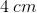. What is the measure of an internal angle?cannot be determined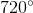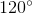Explanation:

Given that the hexagon is a regular hexagon, this means that all the side length are congruent and all internal angles are congruent. The question requires us to solve for the measure of an internal angle. Given the aforementioned definition of a regular polygon, this means that there must only be one correct answer.

In order to solve for the answer, the question provides additional information that isn't necessarily required. The measure of an internal angle can be solved for using the equation: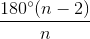whereis the number of sides of the polygon.

In this case,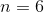.

For this problem, the information about the side length may be negated.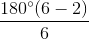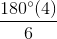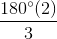### Example Question #901 : Intermediate Geometry

What is the interior angle of a regular hexagon if the area is 15?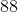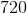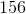Explanation:

The area has no relevance to find the angle of a regular hexagon.

There are 6 sides in a regular hexagon.  Use the following formula to determine the interior angle.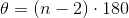Substitutesides to determine the sum of all interior angles of the hexagon in degrees.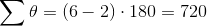Since there are 6 sides, divide this number by 6 to determine the value of each interior angle.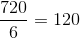### Example Question #1 : How To Find An Angle In A Hexagon

Given: Regular Hexagon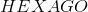with center. Construct segments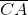and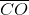to form Quadrilateral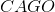.

True or false: Quadrilateralis a rectangle.

False

True

False

Explanation:

Below is regular Hexagonwith center, a segment drawn fromto each vertex - that is, each of its radii drawn.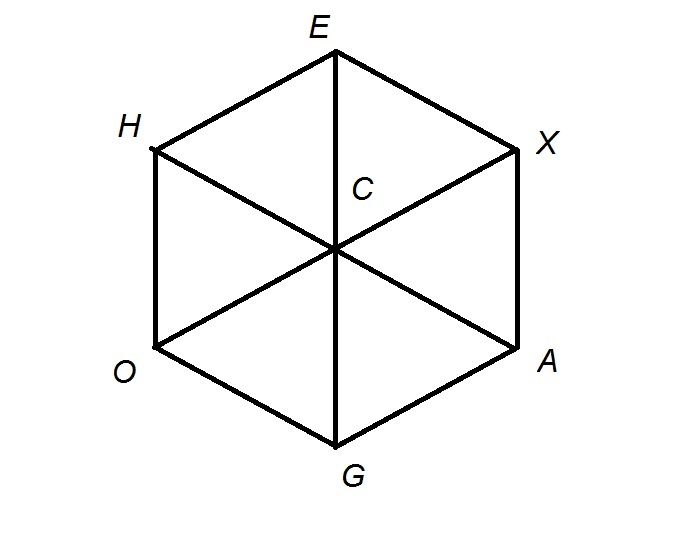Each angle of a regular hexagon measures; by symmetry, each radius bisects an angle of the hexagon, so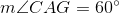.

The angles of a rectangle must measure, so it has been disproved that Quadrilateralis a rectangle.

### Example Question #1 : How To Find An Angle In A Hexagon

True or false: Each of the six angles of a regular hexagon measures.

True

False

True

Explanation:

A regular polygon withsides hascongruent angles, each of which measures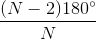Setting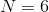, the common angle measure can be calculated to be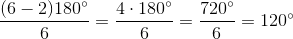The statement is therefore true.

### Example Question #904 : Intermediate Geometry

True or false: Each of the exterior angles of a regular hexagon measures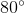.

True

False

False

Explanation:

If one exterior angle is taken at each vertex of any polygon, and their measures are added, the sum is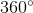. Each exterior angle of a regular hexagon has the same measure, so if we letbe that common measure, then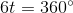Solve for: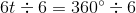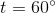The statement is false.

### Example Question #1 : How To Find An Angle In A Hexagon

Given: Hexagon.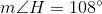True, false, or undetermined: Hexagonis regular.

True

False

Undetermined

False

Explanation:

Suppose Hexagonis regular. Each angle of a regular polygon ofsides has measureA hexagon has 6 sides, so set; each angle of the regular hexagon has measure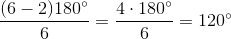Since one angle is given to be of measure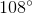, the hexagon cannot be regular.

### Example Question #906 : Intermediate Geometry

What is the measure of one exterior angle of a regular twenty-sided polygon?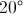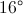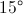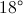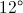Explanation:

The sum of the exterior angles of any polygon, one at each vertex, is. In a regular polygon, the exterior angles all have the same measure, so divide 360 by the number of angles, which, here, is 20, the same as the number of sides.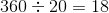### Example Question #1 : How To Find An Angle In A Hexagon

Which of the following cannot be the six interior angle measures of a hexagon?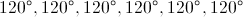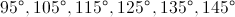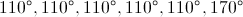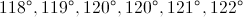All of these can be the six interior angle measures of a hexagon.

All of these can be the six interior angle measures of a hexagon.

Explanation:

The sum of the interior angle measures of a hexagon is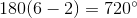Add the angle measures in each group.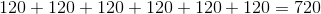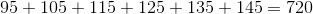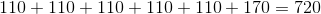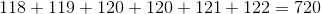In each case, the angle measures add up to 720, so the answer is that all of these can be the six interior angle measures of a hexagon.